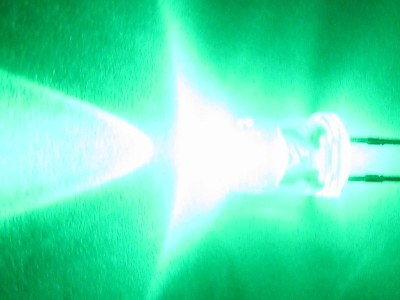# How to calculate current-limiting resistor for LEDsHere is typically how you calculate the “current-limiting resistor” for an LED.

You will need to know ahead of time:

1) Supply voltage Vdd, in case of the typical 5V arduino boards, Vdd=5V

2) Typical forward bias voltage of the LED Vfb, read the spec sheet. My personal experience is that more powerful LEDs have higher value of Vfb.
Take this one for example:

http://dipmicro.com/store/LED5G

A range of 3.0 to 3.6V is given. I would try to use the average of 3.3V. Some other spec sheets give typical values and you will use that value.

3) Current rating i_m. The above LED is said to have 20mA current rating. It’s the maximal sustained current you want it to have. Again some other spec sheets give you current at typical forward bias voltage and you can use that value.

Now to calculate the current-limiting resistor, just do this:

Vdd=Vfb+Vr, where Vr is the voltage dropped on the current-limiting resistor. So Vr=i_m*R. Where i_m is the LED current rating.

So R=(Vdd-Vfb)/i_m

For the above LED, R=(5V-3.3V)/0.02A=85 ohm. That is when you are pushing a little bit. Plus, arduino pins can only supply 20mA and the entire ATMEGA chip can supply 200mA. So to be safe, I would use a 150 ohm resistor. Then the LED will be less bright. If you use the LED for lighting purpose, its brightness is relevant to you. But you use it as an indicator instead of illumination, make it not that bright by using larger resistors.

Typically, if an LED is passing 1mA of current, you can see it in the dark, or straight on in a room but hard to see from sideways. If you run 20mA on an LED, it hurts your eyes to stare at it.

The following is taken in a dark bedroom. I believe only 3 LEDs were on together, passing around 6mA each only 🙂This site uses Akismet to reduce spam. Learn how your comment data is processed.